• 偏导数和导数的区别
2022-03-17 19:51:58

# 机器学习：导数与偏导数

导数和偏导数没有本质区别，都是当自变量的变化趋于0时，函数值的变化量与自变量变化量的比值的极限（如果极限存在的话）。

一元函数，一个y对应一个x，导数只有一个。

二元函数，一个z对应一个x和一个y，有两个导数：一个z对x的导数，一个z对y的导数，也叫做偏导数。

求偏导数时要注意，对一个变量求导，另一个变量视为常数，只对改变量求导，从而将偏导数的求解转化为了一元函数的求解。机器学习
更多相关内容
• 导数定义： 反应的是函数 y=f(x)y=f(x)y=f(x) 在某一点处沿着自变量 xxx 的正方向（即: xxx 轴正方向）的变化率。导数公式：函数 y=f(x)y=f(x)y=f(x) 在 x0x_0x0​ 点的导数记作f′(x0)f'(x_0)f′(x0​)，则 f′(x0)...

# 1.导数

导数定义： 反应的是函数 y = f ( x ) y=f(x) 在某一点处沿着自变量 x x 的正方向（即: x x 轴正方向）的变化率。

导数公式：

函数 y = f ( x ) y=f(x) x 0 x_0 点的导数记作 f ′ ( x 0 ) f'(x_0) ，则 f ′ ( x 0 ) f'(x_0) 为：
f ′ ( x 0 ) = lim ⁡ Δ x → 0 Δ y Δ x = lim ⁡ Δ x → 0 f ( x 0 + Δ x ) − f ( x 0 ) Δ x f' (x_0) = \lim_{\Delta x \to 0} \frac{\Delta y}{\Delta x} = \lim_{\Delta x \to 0} \frac{f(x_0 + \Delta x) - f(x_0)}{\Delta x}

几何意义： 函数 y = f ( x ) y=f(x) x 0 x_0 点的导数 f ′ ( x 0 ) f'(x_0) 表示函数曲线在点 P 0 ( x 0 , f ( x 0 ) ) P_0(x_0, f(x_0)) 处的切线的斜率【导数的几何意义是该函数曲线在这一点 P 0 ( x 0 , f ( x 0 ) ) P_0(x_0, f(x_0)) 上的切线斜率】。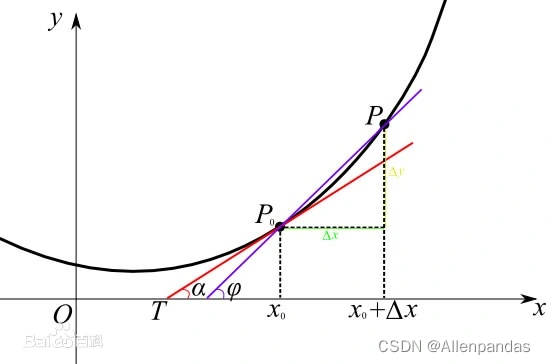# 2.偏导数

偏导数定义： 以二元函数为例，反应的是函数 z = f ( x , y ) z=f(x,y) 在某一点处沿着某个坐标轴正方向（即：沿着 x x 轴正方向或者沿着 y y 轴正方向）的变化率。

偏导数公式：

以二元函数 z = f ( x , y ) z=f(x, y) 为例：
函数 z = f ( x , y ) z=f(x,y) ( x 0 , y 0 ) (x_0, y_0) 点处对 x x 的偏导数记作 ∂ z ∂ x \frac{\partial z}{\partial x} （又可记作： ∂ f ∂ x \frac{\partial f}{\partial x} , z x z_x f x ( x , y ) f_x(x,y) ），则 ∂ z ∂ x \frac{\partial z}{\partial x} 为：

∂ z ∂ x = lim ⁡ Δ x → 0 f ( x 0 + Δ x , y 0 ) − f ( x 0 , y 0 ) Δ x \frac{\partial z}{\partial x} = \lim_{\Delta x \to 0} \frac{f(x_0 + \Delta x, y_0) - f(x_0, y_0)}{\Delta x}

函数 z = f ( x , y ) z=f(x,y) ( x 0 , y 0 ) (x_0, y_0) 点处对 y y 的偏导数记作 ∂ z ∂ y \frac{\partial z}{\partial y} （又可记作： ∂ f ∂ y \frac{\partial f}{\partial y} , z y z_y f y ( x , y ) f_y(x,y) ），则 ∂ z ∂ y \frac{\partial z}{\partial y} 为：

∂ z ∂ y = lim ⁡ Δ y → 0 f ( x 0 , y 0 + Δ y ) − f ( x 0 , y 0 ) Δ y \frac{\partial z}{\partial y} = \lim_{\Delta y \to 0} \frac{f(x_0, y_0 + \Delta y) - f(x_0, y_0)}{\Delta y}

注： 导数与偏导数本质是⼀致的，都是当⾃变量的变化趋于0时，函数值的变化与⾃变量的变化，它们两者之间⽐值的极限。

# 3.方向导数

在前⾯导数偏导数的定义中，均是沿坐标轴正⽅向讨论函数的变化率。那么当我们讨论函数沿任意⽅向的变化率时，也就引出了⽅向导数的定义。

方向导数： 反应的是函数 y y 在某一点 x 0 x_0 处沿着特定方向（不一定是 x x 轴正方向了）的变化率。

# 4.梯度

梯度的提出只为了回答一个问题：函数在变量空间的某一点处，沿着哪个方向有最大的变化率？
梯度的定义如下：函数在某一点的梯度是这样的一个向量，它的方向与最大方向导数的方向一致，而它的大小为方向导数的最大值。

注意：
1）梯度是一个向量，即有方向有大小；
2）梯度的方向就是最大方向导数的方向，即：函数增长最快的方向。
3）梯度的值，就是最大方向导数的值。

区别： 偏导数只能对坐标轴某一方向求导数，方向倒数可以对自变量定义域内任意方向求导，而梯度是方向方向导数值取最大的一个特殊情况。

展开全文机器学习
• ## 导数与偏导

千次阅读 2021-08-22 15:11:49
导数2 偏导数2.1 多变量函数2.2 偏导数3 多变量函数的最小值条件4 参考资料 1. 导数 函数 y=f(x)y=f(x)y=f(x) 的导函数f′(x)f'(x)f′(x)的定义如下所示： 该公式是指，当 ∆x∆x∆x 无限接近0时，f′(x)f'(x)f′...

# 1. 导数

函数 y = f ( x ) y=f(x) 的导函数 f ′ ( x ) f'(x) 的定义如下所示：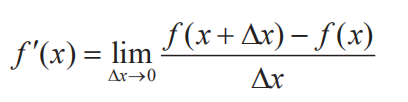该公式是指，当 ∆ x ∆x 无限接近0时， f ′ ( x ) f'(x) 最接近的值是多少。

例如：

f ( x ) = 3 x f(x) = 3x 时，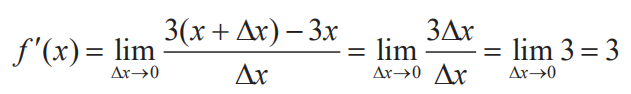f ( x ) = x 2 f (x) = x^2 时，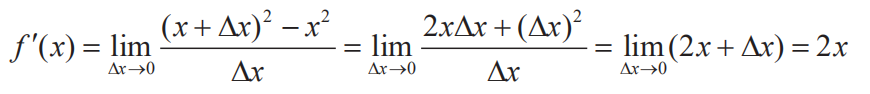常用的求导公式有：
( k ) ′ = 0 、 ( k x ) ′ = k 、 ( x k ) ′ = k x k − 1 、 ( e x ) ′ = e x 、 ( e − x ) ′ = − e − x (k)'=0、(kx)'=k、(x^k)'=kx^{k-1}、(e^x)'=e^x、(e^{-x})'=-e^{-x} （k为常数）

另一种表示方法：
f ′ ( x ) = d y d x f'(x) = \frac{dy}{dx}

由于导函数 f ′ ( x ) f'(x) 表示切线斜率，故：当函数f(x)在x = a处取得最小值时，f’(a) = 0。

# 2 偏导数

## 2.1 多变量函数

有两个以上的自变量的函数称为多变量函数。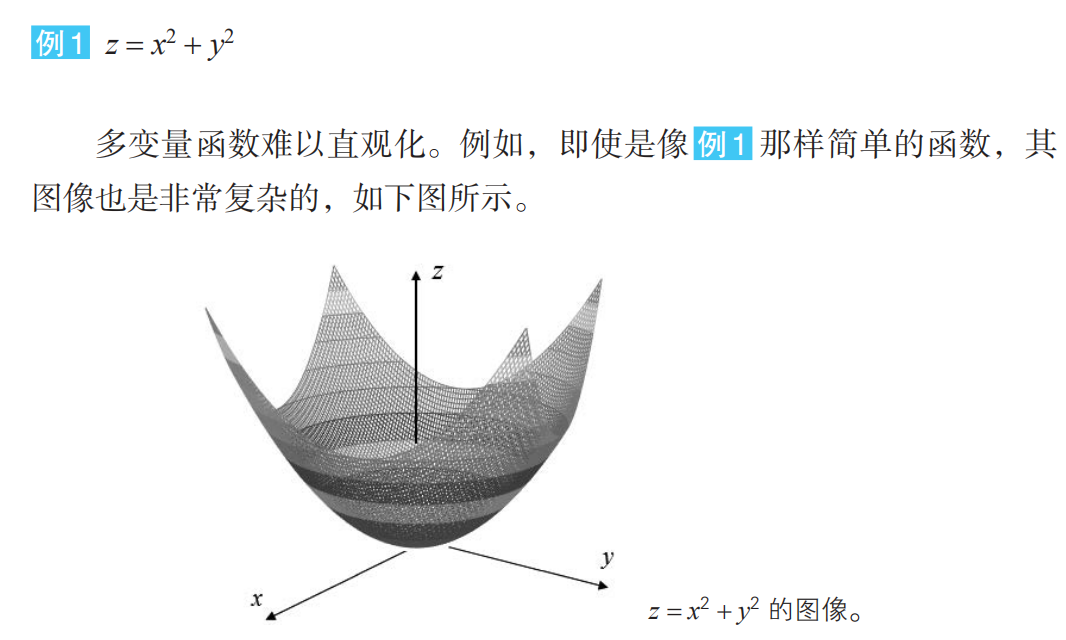## 2.2 偏导数

求导的方法也同样适用于多变量函数的情况。但是，由于有多个变量，所以必须指明对哪一个变量进行求导。在这个意义上，关于某个特定变量的导数就称为偏导数

例如，让我们来考虑有两个变量 x、y 的函数 z = f(x, y)。只看变量 x， 将 y 看作常数来求导，以此求得的导数称为“关于 x 的偏导数”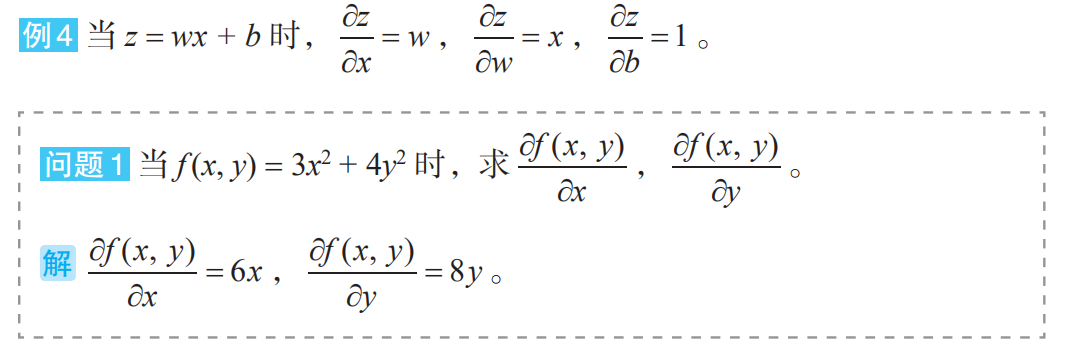# 3 多变量函数的最小值条件

光滑的单变量函数 y = f (x) 在点 x 处取得最小值的必要条件是导函数在该点取值 0，这个事实对于多变量函数同样适用。例如对于有两个变量的函数，可以如下表示。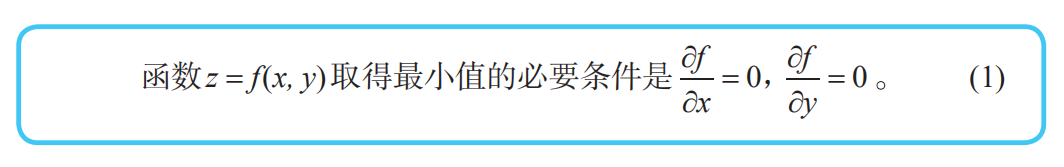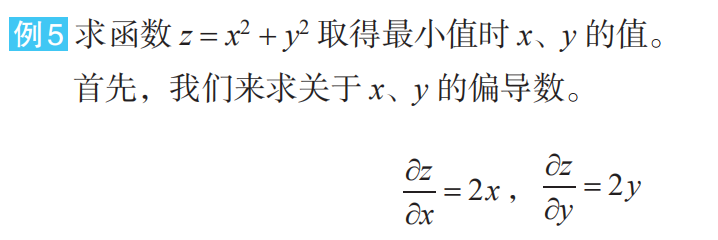根据上述 (1)，函数取得最小值的必要条件是 x = 0，y = 0。此时函数值 z 为 0。由于 z = x 2 + y 2 ≥ 0 z = x^2 + y^2 ≥ 0 ，所以我们知道这个函数值 0 就是最小值。

# 4 参考资料

《深度学习的数学》

展开全文• 前言 　机器学习中的大部分问题都是优化问题，而绝大部分优化问题都可以使用梯度下降法处理，那么搞懂什么是梯度，什么是...　提到梯度，就必须从导数（derivative）、偏导数（partial derivative）方向导数（dir...

转自：https://www.cnblogs.com/lingjiajun/p/9895753.html

前言
机器学习中的大部分问题都是优化问题，而绝大部分优化问题都可以使用梯度下降法处理，那么搞懂什么是梯度，什么是梯度下降法就非常重要！这是基础中的基础，也是必须掌握的概念！
提到梯度，就必须从导数（derivative）、偏导数（partial derivative）和方向导数（directional derivative）讲起，弄清楚这些概念，才能够正确理解为什么在优化问题中使用梯度下降法来优化目标函数，并熟练掌握梯度下降法（Gradient Descent）。

本文主要记录我在学习机器学习过程中对梯度概念复习的笔记，主要参考《高等数学》《简明微积分》以及维基百科上的资料为主，文章小节安排如下：
1）导数
2）导数和偏导数
3）导数与方向导数
4）导数与梯度
5）梯度下降法

导数
一张图读懂导数与微分：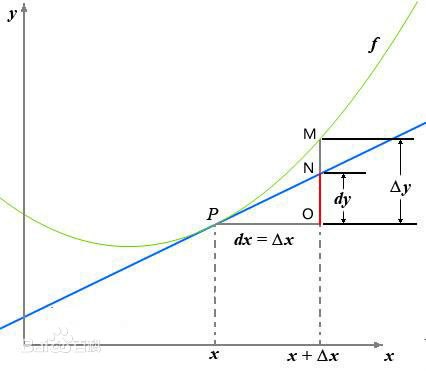这是高数中的一张经典图，如果忘记了导数微分的概念，基本看着这张图就能全部想起来。
导数定义如下：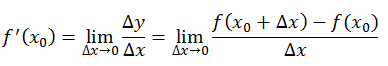反映的是函数y=f(x)在某一点处沿x轴正方向的变化率。再强调一遍，是函数f(x)在x轴上某一点处沿着x轴正方向的变化率/变化趋势。直观地看，也就是在x轴上某一点处，如果f’(x)>0，说明f(x)的函数值在x点沿x轴正方向是趋于增加的；如果f’(x)<0，说明f(x)的函数值在x点沿x轴正方向是趋于减少的。

这里补充上图中的Δy、dy等符号的意义及关系如下：
Δx：x的变化量；
dx：x的变化量Δx趋于0时，则记作微元dx；
Δy：Δy=f(x0+Δx)-f(x0)，是函数的增量；
dy：dy=f’(x0)dx，是切线的增量；
当Δx→0时，dy与Δy都是无穷小，dy是Δy的主部，即Δy=dy+o(Δx).

导数和偏导数
偏导数的定义如下：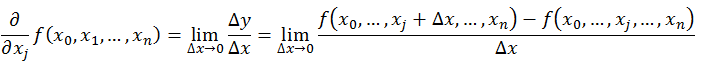可以看到，导数与偏导数本质是一致的，都是当自变量的变化量趋于0时，函数值的变化量与自变量变化量比值的极限。直观地说，偏导数也就是函数在某一点上沿坐标轴正方向的的变化率。
区别在于：
导数，指的是一元函数中，函数y=f(x)在某一点处沿x轴正方向的变化率；
偏导数，指的是多元函数中，函数y=f(x1,x2,…,xn)在某一点处沿某一坐标轴（x1,x2,…,xn）正方向的变化率。

导数与方向导数
方向导数的定义如下：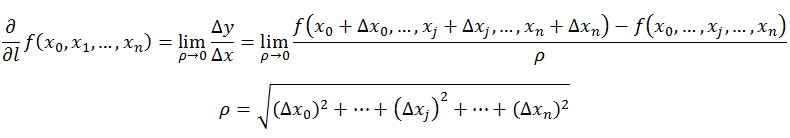在前面导 数和偏导数的定义中，均是沿坐标轴正方向讨论函数的变化率。那么当我们讨论函数沿任意方向的变化率时，也就引出了方向导数的定义，即：某一点在某一趋近方向上的导数值。
通俗的解释是：
我们不仅要知道函数在坐标轴正方向上的变化率（即偏导数），而且还要设法求得函数在其他特定方向上的变化率。而方向导数就是函数在其他特定方向上的变化率。

导数与梯度
梯度的定义如下：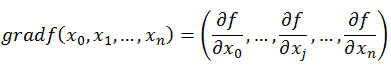梯度的提出只为回答一个问题：
函数在变量空间的某一点处，沿着哪一个方向有最大的变化率？
梯度定义如下：
函数在某一点的梯度是这样一个向量，它的方向与取得最大方向导数的方向一致，而它的模为方向导数的最大值。
这里注意三点：
1）梯度是一个向量，即有方向有大小；
2）梯度的方向是最大方向导数的方向；
3）梯度的值是最大方向导数的值。

导数与向量
提问：导数与偏导数与方向导数是向量么？
向量的定义是有方向（direction）有大小（magnitude）的量。
从前面的定义可以这样看出，偏导数和方向导数表达的是函数在某一点沿某一方向的变化率，也是具有方向和大小的。因此从这个角度来理解，我们也可以把偏导数和方向导数看作是一个向量，向量的方向就是变化率的方向，向量的模，就是变化率的大小。
那么沿着这样一种思路，就可以如下理解梯度：
梯度即函数在某一点最大的方向导数，函数沿梯度方向函数有最大的变化率。

梯度下降法
既然在变量空间的某一点处，函数沿梯度方向具有最大的变化率，那么在优化目标函数的时候，自然是沿着负梯度方向去减小函数值，以此达到我们的优化目标。
如何沿着负梯度方向减小函数值呢？既然梯度是偏导数的集合，如下：同时梯度和偏导数都是向量，那么参考向量运算法则，我们在每个变量轴上减小对应变量值即可，梯度下降法可以描述如下：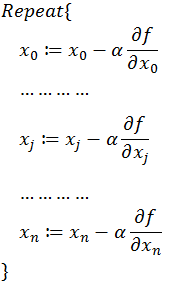总结：

1.导数定义： 导数代表了在自变量变化趋于无穷小的时候，函数值的变化与自变量的变化的比值。几何意义是这个点的切线。物理意义是该时刻的（瞬时）变化率。

注意：在一元函数中，只有一个自变量变动，也就是说只存在一个方向的变化率，这也就是为什么一元函数没有偏导数的原因。
（derivative）

2.偏导数： 既然谈到偏导数，那就至少涉及到两个自变量。以两个自变量为例，z=f（x,y），从导数到偏导数，也就是从曲线来到了曲面。曲线上的一点，其切线只有一条。但是曲面上的一点，切线有无数条。而偏导数就是指多元函数沿着坐标轴的变化率。
注意：直观地说，偏导数也就是函数在某一点上沿坐标轴正方向的的变化率。
（partial derivative）

3.方向导数: 在某点沿着某个向量方向上的方向导数，描绘了该点附近沿着该向量方向变动时的瞬时变化率。这个向量方向可以是任一方向。

方向导数的物理意义表示函数在某点沿着某一特定方向上的变化率。
注意：导数、偏导数和方向导数表达的是函数在某一点沿某一方向的变化率，也是具有方向和大小的。
（directional derivative）

4.梯度: 函数在给定点处沿不同的方向，其方向导数一般是不相同的。那么沿着哪一个方向其方向导数最大，其最大值为多少，这是我们所关心的，为此引进一个很重要的概念: 梯度。

5.梯度下降
在机器学习中往往是最小化一个目标函数 L(Θ)，理解了上面的内容，便很容易理解在梯度下降法中常见的参数更新公式：

Θ = Θ − γ ∂ L ∂ Θ
通过算出目标函数的梯度（算出对于所有参数的偏导数）并在其反方向更新完参数 Θ ，在此过程完成后也便是达到了函数值减少最快的效果，那么在经过迭代以后目标函数即可很快地到达一个极小值。

6.In summary:
概念 　　物理意义
导数 　　函数在该点的瞬时变化率
偏导数 　函数在坐标轴方向上的变化率
方向导数 函数在某点沿某个特定方向的变化率
梯度 　　函数在该点沿所有方向变化率最大的那个方向

展开全文机器学习 梯度
• ## 导数和偏导数

千次阅读 2017-12-11 10:16:15
导数（Derivative）是微积分中的重要基础概念。当函数y=f(x)的自变量X在一点x0上产生一个增量Δx时，函数输出值的增量Δy与自变量增量Δx的...把y固定在y0而让x在x0有增量△x，相应地函数z=f(x,y)有增量(称为对x的
• 偏导数导数 偏导数 由于是二元函数，有两个因变量。偏导数表示分别对某一个导数求导，如偏x导数、偏y导数。 高阶偏导数偏导数继续求导。以二元函数的二阶偏导数为例，偏x导数有两个偏导数、偏y导数有两个偏导数...高等数学 隐函数
• 1、导数 其实求导数是为了方便我们后来求极值，导数的几何意义指该函数曲线在这一点上的切线...如果当△x→ 时，△y/△x 之比的极限存在 则成为 y = f（x）在x₀处可，并且该极限值称为函数f（x）在点x₀处的...
• 导数： 定义： 设函数y= f（x）在点x₀的...如果当△x→ 时，△y/△x 之比的极限存在 则成为 y = f（x）在x₀处可，并且该极限值称为函数f（x）在点x₀处的导数。 f（x）= x² f`(x) = (x + △x)² - x²）/...
• 下边我们将从导数偏导数，方向导数最后引出梯度，进而讲解为什么梯度下降法能够做到求解最优解。 1.1 导数的定义 增量定义： f(x)在点xxx0 的某个邻域内有定义，则当自变量 xxx 在 xxx0处取得增量Δx （点xxx0 + ...机器学习
• 　提到梯度，就必须从导数（derivative）、偏导数（partial derivative）方向导数（directional derivative）讲起，弄清楚这些概念，才能够正确理解为什么在优化问题中使用梯度下降法来优化目标函数，并熟练掌握...机器学习 人工智能 神经网络 python
• 是指多元函数沿坐标轴方向的方向导数，因此二元函数就有两个偏导数。 偏导函数：是一个函数；是一个关于点的偏导数的函数。 方向导数：是一个数；反映的是f(x,y)在点沿方向v的变化率。 梯度：是一个向量；每个...
• 首先，偏导数，人家毕竟带着“导数”二字，想必和导数脱不了干系。事实还真就是脱不了干系。那么，啥是个导数？ 如图所示，这是函数的图像，我们观察这个点，函数图像在这个点的坐标为，我们想像在这个点，x增大...
• ## 全导数、偏导数、方向导数

万次阅读 多人点赞 2018-03-23 00:45:20
导数偏导数、方向导数1、全导数导数本质上就是一元函数的导数。他是针对复合函数而言的定义。 一元函数的情况下，导数就是函数的变化率，从几何意义上看就是：但是在多元的情况下比一元的复杂，下面用二元...
• 该函数用来计算任意函数的一阶偏导数(数值方法),车牌识别定位程序的部分功能，经典的灰度共生矩阵纹理计算方法。
• 文章目录前言正文导数的定义导数的含义及常见函数导数导数的性质分数的求导导数和函数最小值的关系（重点）函数的增减表偏导数多变量函数偏导数的求解（重点）多变量函数的极值问题（重点）躲不掉的拉格朗日总结 ...神经网络
• 由于误差反向传播算法中采用梯度下降算法进行权重更新，因此需要先明白梯度是什么，而梯度的解释又要从导数讲起，因此本文先大致讲解一下导数导数偏导数、方向导数和梯度的物理意义。 1、导数 根据我们以前的学习...随机梯度下降 人工智能 神经网络
• ## 理解偏导数、梯度、方向导数

千次阅读 多人点赞 2020-06-11 22:24:59
偏导数是针对多变量函数而言的，它通过将多变量函数退化成一元函数分别求各自的导数。以二元函数为例： Z = F（x，y） 求x的偏导数就是将y变量看成常量，然后对x求导。 总结：偏导数为函数在每个位置处沿着自变量...
• 导数 导数（Derivative），也叫导函数值。又名微商，是微积分中的重要基础概念。当函数y=f（x）的自变量x在一点x0上产生一个增量Δx时，函数输出值的增量...于是偏导数就出现了，它将多元函数中的一个元看作变量，其余梯度
• 导数的几何意义及代码实现，偏导数定义及几何意义，梯度定义及代码实现，梯度下降法求函数最小值的实现过程python 神经网络
• 可以看出偏导数和方向导数从本质上是一个性质，都是描述某个位置的自变量变化率。只不过偏导数的方向平行某个维度，而方向导数的方向是任意的（偏导数是特殊的方向导数？）。梯度指向的就是变化率（增长）最大的那一...
• 包括全微分、偏导数、方向导数、梯度、全导数等内容。初学这些知识的时候，学生会明显觉得这些概念不难掌握，而且定义及计算公式也很容易记住，但总觉得差那么点东西，说又不知道从何说起。反正笔者是这种感觉。其实...
• 偏导数：函数（因变量对应实数值） 梯度：向量（向量的每一维对应偏导数） 方向导数：函数（因变量对应实数值） 梯度下降：一种优化方法 二阶导数：函数（因变量对应实数值） 二阶方向导数：函数（因变量对应实...机器学习 人工智能
• 学习到机器学习线性回归逻辑回归时遇到了梯度下降算法，然后顺着扯出了一堆高数的相关概念理论：导数偏导数、全微分、方向导数、梯度，重新回顾它们之间的一些关系，从网上教材中摘录相关知识点。 这段是我...
• 参考博客https://blog.csdn.net/baishuo8/article/details/81408369知乎https://www.zhihu.com/question/36301367 一、导数（derivative） 导数，是我们最早接触的一元函数中定义的，可以在 xy 平面直角坐标...
• 一、隐函数 显函数：解析式中明显地用一个变量的代数式表示另一个变量时，称为显函数。显函数可以用y=f(x)来表示。...隐函数与显函数的区别： 　1） 隐函数不一定能写为y=f(x)的形式，如x²+y...
• 包括全微分、偏导数、方向导数、梯度、全导数等内容。 一、导数和微分到底是什么，以及为什么会有这些概念 导数和微分其实就是数学家创造的两个代数工具，是为了从代数的角度来描述函数图像在几何上的变化。...
• 偏导数、微分、以及导数到底有什么关系和区别？ https://www.zhihu.com/question/265021971
• @梯度 梯度 为什么梯度是函数方向...偏导数==>方向导数==>梯度由浅入深的分析梯度 提示：以下是本篇文章正文内容，下面案例可供参考 一、导数 二、偏导数 三、偏导数几何意义 四、方向导数与梯度 ...机器学习 python
• 在一个多变量的函数中，偏导数就是关于其中一个变量的导数而保持其他变量恒定不变。 假定二元函数z=f(x,y)z=f(x,y)z=f(x,y), 点(x_0, y_0)是其定义域内的一个点，将y固定在y0y_0y0​上，而x在x0x_0x0​上增量△x\...AI...